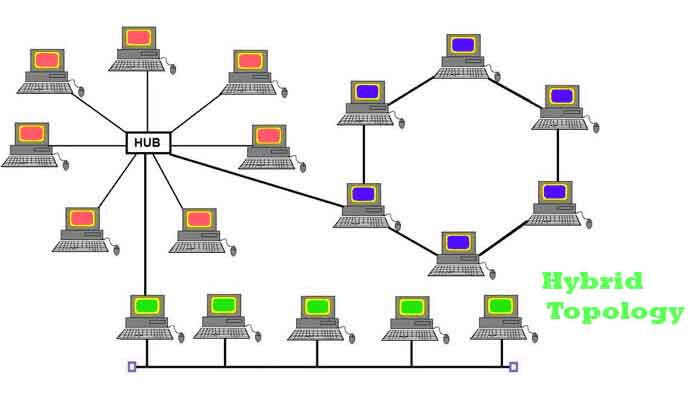Simply the physical layout or logical layout of a structure is called Topology. It is in the topology, where are the nodes are connected together. It is the arrangement of nodes or elements in the computer network is called a Network topology. We have different types of topologies like Bus, Ring, Mesh, start, and Tree topology. Let us discuss the Two Examples Of Hybrid Topologies.

The below diagram is the structure of the Hybrid topology. We can also be called the star wired Bus topology. Different star topologies are connected through a Bus topology. It is the combined structure of two different topologies. The Hybrid topology is also called the tree Topology. The Hybrid topology allows for the expansion of the existing network. It is only used when flexibility is required in the network.

There is a difference in the tree topology, the combination of the ring and star becomes the Hybrid topology but not as the Tree topology. The below diagram represents the ring and starts topology which doesn’t be called the tree topology.The partial Mesh and the extended star are the Two Examples Of Hybrid Topologies. The partial Mesh topology is not a connection to every computer network. Not all the site networks are connected to all the networks in an area. It is called the partial Mesh topology.

A full mesh can give an optimal path for better communication but it is so expensive. The Partial Mesh is the subset of a full Mesh, it is the strategically placed links where it is most needed. The Extended star topology is the best solution for the organizations less of a single point of failure.

Also Read:

It is more flexible than the star topology, the one point of a network cannot cause the entire network to fail at any possible time, but if it affected the center of the star hub, it affects most of the devices connected to the center hub.